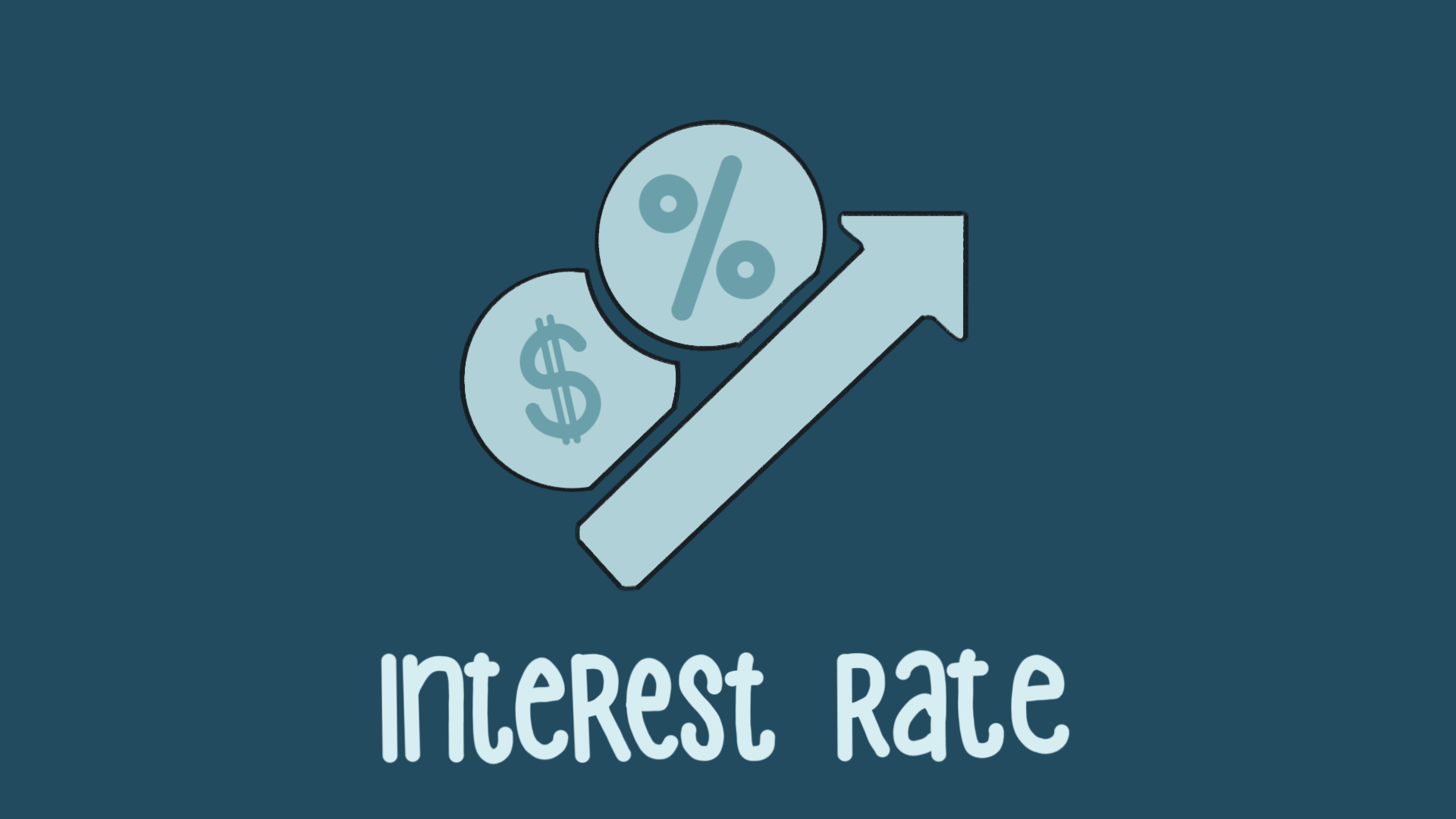Interest RatesThe term “interest rate” is one of the most commonly used phrases in a fixed-income investment lexicon. Different types of interest rates, including real, nominal, effective and annual, are distinguished by key economic factors, which can help individuals become smarter consumers and shrewder investors. Here's a breakdown of these interest rates and how each might impact consumers seeking credit or a loan.

Nominal Interest Rate

The nominal interest rate is the stated rate of interest of a bond or loan, which signifies the actual monetary price borrowers pay lenders to use their money. If the nominal rate on a loan is 5%, borrowers can expect to pay \$5 of interest for every \$100 loaned to them. This is often referred to as the coupon rate because it was traditionally stamped on the coupons redeemed by bondholders.

Real Interest Rate

Real interest rates, unlike nominal rates, take account of inflation, to offer investors a more accurate measure of their buying power after they redeem their positions. If an annually-compounding bond lists a 6% nominal yield and the inflation rate is 4%, then the real rate of interest is actually only 2%. It’s feasible for real interest rates to be in negative territory if the rate of inflation exceeds the nominal rate of an investment. For instance, a bond with a third nominal rate will have a real interest rate of -1%, if the inflation rate is 4%. A comparison of real and nominal interest rates can be calculated using this equation:

Real Rate of Return = Nominal Interest Rate − Inflation Rate

Several economic stipulations can be derived from this formula, which lenders, borrowers, and investors may utilize to cultivate more informed financial decisions. Typically, when the inflation rates are negative (deflationary), real rates exceed nominal rights. But the opposite is true when inflation rates are positive.

One theory suggests the inflation rate moves in tandem with nominal interest rates over time, meaning that real interest rates become stable over long time periods. Therefore, investors with longer time horizons could be able to more accurately assess their investment returns on an inflation-adjusted basis.

Effective Interest Rate

Investors and borrowers should also be conscious of the effective interest rate, which takes the concept of compounding under consideration. For instance, if a bond pays 6% annually and compounds semi-annually, an investor who places ₹100,000 in this bond will receive ₹3000 of interest payments after the first 6 months (₹100,000 x 0.03) and ₹3090 of interest after the next six months (₹103,000 x 0.03). In total, this investor receives ₹6090 for the year. In this scenario, while the nominal rate is 6%, the effective rate is 6.09%.

Mathematically speaking, the difference between the nominal and effective rates increases with the number of compounding periods within a specific period of time. Note that the principles concerning calculating and advertising the annual equivalent rate (AER) on financial products are less stringent than the principles governing the annual percentage rate (APR).

Applications In Our World

The differences between nominal, real, and effective rates are important when it involves loans. For instance, a loan with frequent compounding periods is going to be more expensive than one that compounds annually, which is a vital consideration when buying mortgages.

Furthermore, a bond that pays just a 1% real interest rate may not adequately grow an investor’s assets over time. Simply put: interest rates effectively reveal the true return that will be posted by a fixed-income investment and therefore the true cost of borrowing for individuals or businesses.

Investors who seek protection from inflation in the fixed-income arena may elect to contemplate Treasury Inflation-Protected Securities (TIPS), which pay an interest rate that is indexed to inflation. Mutual funds investing in bonds, mortgages and senior secured loans that pay floating interest rates, also periodically adjust with current rates.

The Crux

When it involves a bond’s interest rates, shrewd investors know to look beyond nominal or coupon rates when considering their overall investment objectives. A professional financial advisor can help investors navigate interest rates that continue with inflation.

If you dig down into the 'interest' landscape, you will see that there are multiple kinds of interest that may confront a borrower. Thus, it's in the best interest of a borrower to get to know the various kinds of interest and how each may impact the acquisition of credit or a loan.

After all, the more knowledge gained from a better understanding of interest and how it works in all of its forms can be leveraged to get you a better deal the following time you apply for a loan or a credit account.